### IMO Shortlist 2009 problem G3

Kvaliteta:
Avg: 0.0
Težina:
Avg: 7.0
Let$ABC$ be a triangle. The incircle of$ABC$ touches the sides$AB$ and$AC$ at the points$Z$ and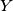$Y$, respectively. Let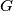$G$ be the point where the lines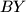$BY$ and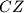$CZ$ meet, and let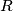$R$ and$S$ be points such that the two quadrilaterals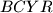$BCYR$ and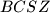$BCSZ$ are parallelogram.
Prove that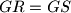$GR=GS$.

Proposed by Hossein Karke Abadi, Iran
Source: Međunarodna matematička olimpijada, shortlist 2009